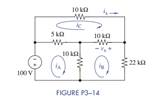### Create an Account

Already have account?

### Forgot Your Password ?

Home / Questions / Formulate mesh-current equations for the circuit in Figure P3 14 Arrange the results in ma...

# Formulate mesh-current equations for the circuit in Figure P3 14 Arrange the results in matrix form Ax = b Solve for iA iB and iCUse these results to find vx and

(a) Formulate mesh-current equations for the circuit in Figure P3−14. Arrange the results in matrix form Ax = b.

(b) Solve for iA, iB, and iC.

(c) Use these results to find vand ix.Jun 15 2020 View more View Less

#### Answer (Solved)Subscribe To Get Solution